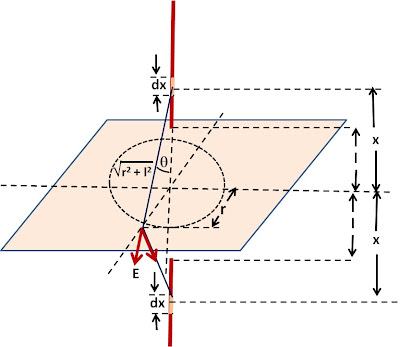## Sunday, November 14, 2010

### Irodov Problem 3.61Following the method of images we replace the infinite plane conductor by a negatively charged rod placed at the mirror image location of the conductor as shown in the figure.

Now let us determine the electric field due to the infintely long charged rods at a location that is at a distance r on the plane of theconductor from its axis as shown in the figure.
Consider an infinitesimally small section of the rod of length dx, at a height x from the plane. The electric field due to this infinitesimally small section of the rod will be,

Thus,

As described in Problem 3.59, the charges induced in the conductor at this location can be determined as,1.2.It's dimensionally incorrect

1.the problem has been rectified in the following steps.

2.dimensionally correct

3.is it necessary for the plate to have an infinite area to apply the method of images.

4.No it's dementionaly correct!

1.